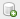[Go to Previous] [Go to Next]

Description of Formulas - Maps

The following describes each formula included in the Maps Formulas section of McIDAS-V.

Note: If the formula selected utilizes a Select Input window to enter values to be used by the formula, the user can choose if to save these values in a bundle if one is created. Each field of the Select Input window has a Save in Bundle option (chosen by default). If this option selected, the value(s) chosen will be stored in the bundle, and if all values are saved, the formula will automatically execute when the bundle is loaded without any user input. If these values are not saved in the bundle, the values used will not be saved, and the formula will not automatically execute and require the user to enter/change values.

• 3D Map (MapData, Topography) - Creates a 3D map over the selected topography field. To utilize this formula, there must be both a map and a topography data source loaded into the Field Selector. One way to create a map data source is by using the Load this map as a data source button () from the Map Controls. One way to create a topography data source is by loading in forecast model data which includes a 2D field such as 'Geopotential height @ Ground or water surface'.
• Average from map (Field, Map) - Plots the average of the selected field with respect to the chosen map.
• Inverse subset field from map (Field, Map) - Plots the values on the map, multiplied by -1, to represent the inverse values for the selected variables/times.
• Make field from map bounds (Number Points X, Number Points Y, Fill Value, Unit) - Makes a field in which the area is the bounds of the given mapSet. The length of the map is determined by the number of points in the X and Y direction.  Input Parameter Definition Number Points X Number of points in the X direction (default=100) Number Points Y Number of points in the Y direction (default=100) Fill Value Fill value (default=0) Unit Unit data will display in
• Subset field from map (Field, Map) - Subsets a display of the chosen field to the map that was loaded in separately as a shapefile.

[Go to Previous] [Go to Next]